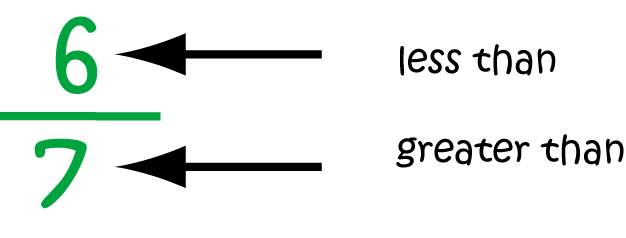# Definition of Proper FractionA proper fraction is a fraction in which the numerator is less than the denominator.

For example,

• $\dfrac{5}{7}$ is a proper fraction since $5 • \(\dfrac{11}{23}$ is a proper fraction since $11 • \(\dfrac{6}{6}$ is not a proper fraction (it is improper), and neither is $\dfrac{23}{11}$.

### Description

The aim of this dictionary is to provide definitions to common mathematical terms. Students learn a new math skill every week at school, sometimes just before they start a new skill, if they want to look at what a specific term means, this is where this dictionary will become handy and a go-to guide for a student.

### Audience

Year 1 to Year 12 students

### Learning Objectives

Learn common math terms starting with letter P

Author: Subject Coach
You must be logged in as Student to ask a Question.